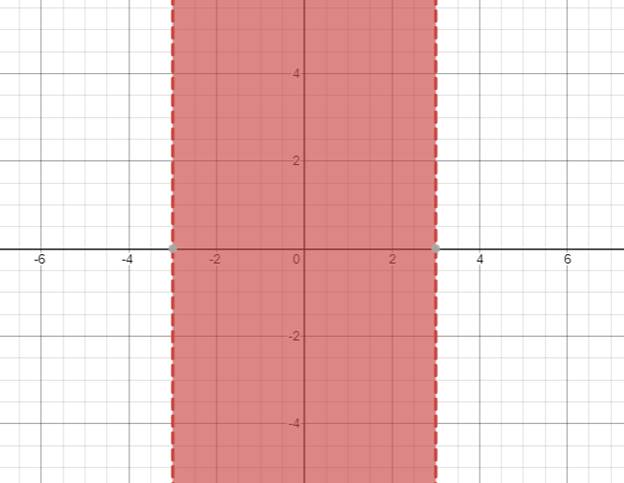# The solution of given nonlinear inequality equation and plot a graph for it.### Precalculus: Mathematics for Calcu...

6th Edition
Stewart + 5 others
Publisher: Cengage Learning
ISBN: 9780840068071### Precalculus: Mathematics for Calcu...

6th Edition
Stewart + 5 others
Publisher: Cengage Learning
ISBN: 9780840068071

#### Solutions

Chapter 1.7, Problem 48E
To determine

## The solution of given nonlinear inequality equation and plot a graph for it.

Expert Solution

3x3

### Explanation of Solution

Given:

Non-linear inequality equation

x29

Calculation,

Non-linear inequality equation,

x29x290x2320(x+3)(x3)0Now,x+30x3andx30x3so,3x3

Now, graph of x29Conclusion:

Hence, the values of x lies between the -3 and 3.

### Have a homework question?

Subscribe to bartleby learn! Ask subject matter experts 30 homework questions each month. Plus, you’ll have access to millions of step-by-step textbook answers!